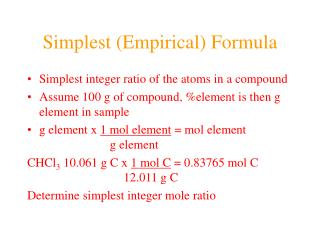DownloadDownload PresentationSimplest (Empirical) Formula

# Simplest (Empirical) Formula

Download Presentation## Simplest (Empirical) Formula

- - - - - - - - - - - - - - - - - - - - - - - - - - - E N D - - - - - - - - - - - - - - - - - - - - - - - - - - -
##### Presentation Transcript

1. Simplest (Empirical) Formula • Simplest integer ratio of the atoms in a compound • Assume 100 g of compound, %element is then g element in sample • g element x 1 mol element = mol element g element CHCl3 10.061 g C x 1 mol C = 0.83765 mol C 12.011 g C Determine simplest integer mole ratio

2. EXAMPLE: Phosphorus burns in air to produce a white compound that is 43.7% P and 56.3% O by mass. What is the empirical formula of the compound? mass 43.7g P 56.3g O Assume 100 g of compound Relative Number of Atoms Multiply by Integer Divide by Smaller (mass/atomic mass) 2  1.00 2 2  2.50 5 43.7/30.97 = 1.41 56.3/15.9994 = 3.52 1.41/1.41 = 1.00 3.52/1.41 = 2.50 .25, .33, .5, .67, .75 ¼, ⅓, ½, ⅔, ¾ 1:1.25 = 4:5 Empirical Formula P2O5

3. Molecular Formula • The exact proportions of the elements that are contained in a molecule • An integer multiple (X) of the empirical formula MF = X  EF

4. Molecular Formula from Simplest Formula empirical formula mass  FM sum of the atomic weights represented by the empirical formula molar mass = MM = X  FM

5. Molecular Formula from Simplest Formula first, knowing MM and FM X = MM/FM then MF = X  EF

6. EXAMPLE: Our phosphorus compound has a molar mass of ~285. What is the molecular formula? FM = 2 x 30.97 + 5 x 16.00 = 141.94 MM 285 X = = = 2 FM 141.94 thus MF = 2 EF P4O10

7. 130 10 0 0 The empirical formula of a substance is found to be CH3O and its molecular weight is found to be roughly 61 g/mol. What is the true molecular weight of the substance? • 30.5 g/mol • 31.0 g/mol • 61.0 g/mol • 62.0 g/mol • 124.0 g/mol

8. Biological Periodic Table

9. Glucose C6H12O6 Carbohydrates Cx(H2O)y

10. Sucrose Glucose + Fructose  Sucrose + Water

11. Chapter 4 Quantities of Reactants and Products

12. Balanced Chemical Equation • Representation of a chemical reaction which uses stoichiometric coefficients (prefix numbers) to represent the relative amounts of reactants and products • 2 H2 (g) + O2 (g)  2 H2O (l) • Molecule to Moleculeor Mole to Mole

13. EXAMPLE How much H2O, in moles results from burning an excess of H2 in 3.3 moles of O2? 2 H2 + O2 2 H2O (3.3 mol O2) (2 mol H2O) #mol H2O = = 6.6 mol H2O (1 mol O2) Mole ratio from balanced chemical equation

14. Reaction of H2 and Cl2 H2 (g) + Cl2 (g)  2 HCl (g) one to one gives two or four to four gives eight

15. Types of Reactions • synthesis or combination reactions • decomposition reactions • displacement reactions • exchange reactions

16. Types of Chemical Reactions

17. Synthesis or Combination Reactions Formation of a compound from simpler compounds or elements.

18. Combination Reaction

19. Decomposition Reactions Separation into constituents by chemical reaction.

20. Dynamite

21. Electrolysis

22. Displacement Reactions Reaction of a compound with a more reactive element to produce a new compound and release a less reactive element

23. Displacement Reactions

24. Exchange Reactions Reaction where ion partners are exchanged

25. Pb(NO3)2 (aq) + K2CrO4 (aq)  PbCrO4 (s) + 2 KNO3 (aq)

26. 130 10 0 0 When Zn(s) is placed in aqueous HCl, hydrogen gas is evolved and zinc chloride solution is obtained. Predict the reaction type. • Combination • Combustion • Decomposition • Displacement (single displacement) • Exchange (double displacement)

27. Writing and BalancingChemical Equations • Determine the type of reaction and formulae of the products • Write an unbalanced equation with the correct reactants and products • Balance the equation by the use of prefixes (coefficients) to balance the number of each type of atom on the reactant and product sides of the equation.

28. Example Iron is produced by the reduction of iron(III) oxide with CO which forms CO2 iron(III) oxide + carbon monoxide  iron + carbon dioxide Fe2O3 + CO  Fe + CO2 Fe2O3 + CO  2 Fe + CO2 Fe2O3 + 3 CO  2 Fe + 3 CO2

29. 130 10 0 0 When aluminum reacts with sulfuric acid to yield aluminum sulfate and hydrogen what is the SUM of the coefficients in the balanced equation? • 4 • 6 • 7 • 8 • 9